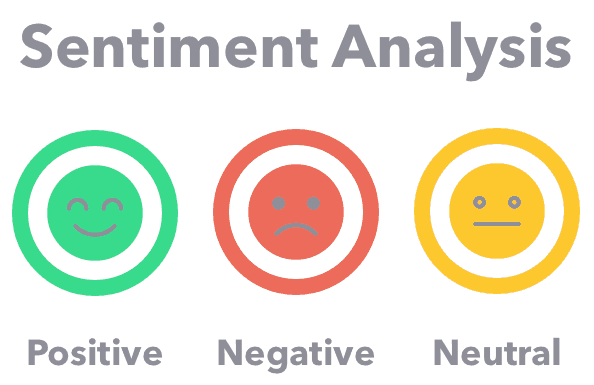# Sentiment analysis of amazon review data using LSTM

Original article was published by Sameer Bairwa on Deep Learning on Medium# Sentiment analysis of amazon review data using LSTM

Hey Folks, we are back again with another article on the sentiment analysis of amazon electronics review data.

So, we have processed amazon review data.

Let’s have a look at it.

If you want to see the pre-processing steps that we have done in the previous article you can check out https://medium.com/@sameerbairwa07/sentiment-analysis-of-amazon-product-reviews-93437ad76b59

So we have the review, rating, sentiment for further process.

Now we will do some more pre-processing for tokenization.
1. remove spacial characters
3. remove stop words

Stop Words: A stop word is a commonly used word (such as “the”, “a”, “an”, “in”) that a search engine has been programmed to ignore, both when indexing entries for searching and when retrieving them as the result of a search query.

{‘ourselves’, ‘hers’, ‘between’, ‘yourself’, ‘but’, ‘again’, ‘there’, ‘about’, ‘once’, ‘during’, ‘out’, ‘very’, ‘having’, ‘with’, ‘they’, ‘own’, ‘an’, ‘be’, ‘some’, ‘for’, ‘do’, ‘its’, ‘yours’, ‘such’, ‘into’, ‘of’, ‘most’, ‘itself’, ‘other’, ‘off’, ‘is’, ‘s’, ‘am’, ‘or’, ‘who’, ‘as’, ‘from’, ‘him’, ‘each’, ‘the’, ‘themselves’, ‘until’, ‘below’, ‘are’, ‘we’, ‘these’, ‘your’, ‘his’, ‘through’, ‘don’, ‘nor’, ‘me’, ‘were’, ‘her’, ‘more’, ‘himself’, ‘this’, ‘down’, ‘should’, ‘our’, ‘their’, ‘while’, ‘above’, ‘both’, ‘up’, ‘to’, ‘ours’, ‘had’, ‘she’, ‘all’, ‘no’, ‘when’, ‘at’, ‘any’, ‘before’, ‘them’, ‘same’, ‘and’, ‘been’, ‘have’, ‘in’, ‘will’, ‘on’, ‘does’, ‘yourselves’, ‘then’, ‘that’, ‘because’, ‘what’, ‘over’, ‘why’, ‘so’, ‘can’, ‘did’, ‘not’, ‘now’, ‘under’, ‘he’, ‘you’, ‘herself’, ‘has’, ‘just’, ‘where’, ‘too’, ‘only’, ‘myself’, ‘which’, ‘those’, ‘i’, ‘after’, ‘few’, ‘whom’, ‘t’, ‘being’, ‘if’, ‘theirs’, ‘my’, ‘against’, ‘a’, ‘by’, ‘doing’, ‘it’, ‘how’, ‘further’, ‘was’, ‘here’, ‘than’}

`from keras.preprocessing.text import Tokenizerfrom keras.preprocessing.sequence import pad_sequences`

Now we will Keras tokenizer to make tokens of words.

`MAX_NB_WORDS = 500000              #vary absed on size of dataset# Max number of words in each complaint.MAX_SEQUENCE_LENGTH = 250# This is fixed.EMBEDDING_DIM = 15tokenizer = Tokenizer(num_words=MAX_NB_WORDS, filters='!"#\$%&()*+,-./:;<=>?@[\]^_`{|}~', lower=True)tokenizer.fit_on_texts(df['reviewFinal'].values)word_index = tokenizer.word_index`

The maximum number of words depends on size of dataset.

Here we split the dataset in 80, 20 ratio

Training on 80000
Testing on 20000

Now let’s define a simple LSTM for training.

`from keras.models import Sequentialfrom keras.layers import Input, Dense, Embedding, SpatialDropout1D, add, concatenatefrom keras.layers.recurrent import LSTMfrom keras.callbacks import ModelCheckpoint, EarlyStoppingmodel = Sequential()model.add(Embedding(MAX_NB_WORDS, EMBEDDING_DIM, input_length=X.shape))model.add(SpatialDropout1D(0.2))model.add(LSTM(15, dropout=0.2, recurrent_dropout=0.2))model.add(Dense(3, activation='softmax'))model.compile(loss='categorical_crossentropy', optimizer='adam', metrics=['accuracy'])epochs = 15batch_size = 32history = model.fit(X_train, Y_train, epochs=epochs, batch_size=batch_size,validation_split=0.1,callbacks=[EarlyStopping(monitor='val_loss', patience=3, min_delta=0.0001)])`

The batch size is 32 and epochs are 15 for training.

now you might be thinking about why it stopped at 5 because the model val_accuracy stopped increasing after 5 epochs.

Evaluation of Model:

`accr = model.evaluate(X_test,Y_test)print('Test set\n  Loss: {:0.3f}\n  Accuracy: {:0.3f}'.format(accr,accr))`

Plotting accuracy curve and confusion matrix

`import matplotlib.pyplot as plt%matplotlib inline#plotting curves for LSTMprint(history.history.keys())#  "Accuracy"plt.plot(history.history['accuracy'])plt.plot(history.history['val_accuracy'])plt.title('model accuracy')plt.ylabel('accuracy')plt.xlabel('epoch')plt.legend(['train', 'validation'], loc='upper left')plt.show()# "Loss"plt.plot(history.history['loss'])plt.plot(history.history['val_loss'])plt.title('model loss')plt.ylabel('loss')plt.xlabel('epoch')plt.legend(['train', 'validation'], loc='upper left')plt.show()`

Accuracy Matix

`def modelEvaluation(predictions, y_test_set):    #Print model evaluation to predicted result     print ("\nAccuracy on validation set: {:.4f}".format(accuracy_score(y_test_set, predictions)))    #print "\nAUC score : {:.4f}".format(roc_auc_score(y_test_set, predictions))    print ("\nClassification report : \n", metrics.classification_report(y_test_set, predictions))    print ("\nConfusion Matrix : \n", metrics.confusion_matrix(y_test_set, predictions))#making predictions using LSTMy_hat = model.predict(X_test)y_hat_class = model.predict_classes(X_test)y_pred_list = y_hat_class.tolist()y_test = []for i in Y_test:    y_test.append(np.argmax(i))modelEvaluation(y_pred_list,y_test)`